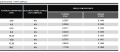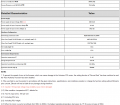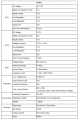# How to estimate Apparent Power

#### PsySc0rpi0n

Joined Mar 4, 2014
1,682
Hello.

I would like to know if it is possible and easily done, to estimate what Apparent Power I need to hire from my Electricity provider in order to be able to have a specific machine working at home of a given power rate, along with other domestic appliances without my Electric board shuts down due to overload.

For instance, if I want to estimate the Apparent Power I need in order to be able to have a 3200Watt machine working and be able to have, for instance, my oven and one other appliance on at the same time ON, how can I estimate the Apparent Power?

My Electricity provider gives us a few values we can hire for Apparent Power. 3.45kVA, 4.6kVA, 6kVA up to at least 10kVA.

How could I estimate?

#### DickCappels

Joined Aug 21, 2008
9,512
Simple:
1) Measure the RMS voltage,
2) Measure the RMS current, and
3) Multiply them to get the apparent power.

#### Ian0

Joined Aug 7, 2020
6,702
Hello.

I would like to know if it is possible and easily done, to estimate what Apparent Power I need to hire from my Electricity provider in order to be able to have a specific machine working at home of a given power rate, along with other domestic appliances without my Electric board shuts down due to overload.

For instance, if I want to estimate the Apparent Power I need in order to be able to have a 3200Watt machine working and be able to have, for instance, my oven and one other appliance on at the same time ON, how can I estimate the Apparent Power?

My Electricity provider gives us a few values we can hire for Apparent Power. 3.45kVA, 4.6kVA, 6kVA up to at least 10kVA.

How could I estimate?
Do you really get charged for reactive power on a domestic supply?
What is the maximum current of your supply?

#### PsySc0rpi0n

Joined Mar 4, 2014
1,682
Simple:
1) Measure the RMS voltage,
2) Measure the RMS current, and
3) Multiply them to get the apparent power.
You mean for each device I have powered on at the same time, sum them and do that math?
I live in EU, so, our mains is 230V, 50Hz. Not sure this helps.

Do you really get charged for reactive power on a domestic supply?
What is the maximum current of your supply?
Yes, there are small differences .

This is just an example of a table of prices according to the kVA I hire.To these values, adds the tax we call IVA, which is 23% on top of those values! Not sure how this tax is called in other languages.

#### DickCappels

Joined Aug 21, 2008
9,512
If you had a way to measure the phase shift between current and voltage then you could intelligently add the currents together and multiply by the voltage (assuming they all see the same voltage.

Lacking that I would calculate the apparent power for each device and then sum up the apparent powers.

You wrote:
You mean for each device I have powered on at the same time, sum them and do that math?
I live in EU, so, our mains is 230V, 50Hz. Not sure this helps.

Its the same procedure at all voltages and frequencies.

#### Ian0

Joined Aug 7, 2020
6,702
Power factor of an induction motor on full load might be as good as 0.9, probably better than 0.8, and 0.9 gives about 1.5kVAR. It’s hardly any worse than your vacuum cleaner.
Just get some power factor,correction capacitors.

I assume IVA is VAT in English or TVA in French

#### PsySc0rpi0n

Joined Mar 4, 2014
1,682
If you had a way to measure the phase shift between current and voltage then you could intelligently add the currents together and multiply by the voltage (assuming they all see the same voltage.

....
You mean a scope? I have one.

Lacking that I would calculate the apparent power for each device and then sum up the apparent powers.
So, if that is the case, and for the example machine I gave of 3200Watt of power, I would have to divide 3200 by 230 to find RMS current. Am I right? And do the same for the other appliances? And after all those summed I would have to multiply it by 230V?

I bet it can't be that simple.

Power factor of an induction motor on full load might be as good as 0.9, probably better than 0.8, and 0.9 gives about 1.5kVAR. It’s hardly any worse than your vacuum cleaner.
Just get some power factor,correction capacitors.

I assume IVA is VAT in English or TVA in French
Yeah, that's it VAT.

And this 3200 Watt machine is not a motor. It's an ASIC device.

#### Ya’akov

Joined Jan 27, 2019
6,856
Are you mining crypto? The power supplies should have labeling in kVA and power factor might be available from the manufacturer.

#### PsySc0rpi0n

Joined Mar 4, 2014
1,682
Are you mining crypto? The power supplies should have labeling in kVA and power factor might be available from the manufacturer.
I'm not mining yet, but that would be the idea, yes. I still have no unit with me, so I can't check that by myself. Let me see if I can find anything in the device characteristics.

Edited;

These are the characteristics of the deviceAnd there are the characteristics of the power supply:Last edited:

#### Ya’akov

Joined Jan 27, 2019
6,856

#### PsySc0rpi0n

Joined Mar 4, 2014
1,682
I assume I should be able to find the Apparent Power from the Correction Factor?
I am not anywhere near to be familiar with the math. I never engaged with mains and never understood it correctly, so I'm a bit lost!

I know that PF = True Power / Apparent Power
But I'm never sure, when we need to calculate these Powers, which values to take into consideration.
Could I simply do something like 0.99 = 3068Watt / x <=> x = 3068 / 0.99 = 3098.98VA ~ 3.1kVA ?

#### Ya’akov

Joined Jan 27, 2019
6,856
It's so close to 1 that you can assume is it acting like a resistive load and just ignore the PF. If you are pushing things so close that < .01 PF matters, you are going to have problems anyway.

#### PsySc0rpi0n

Joined Mar 4, 2014
1,682
It's so close to 1 that you can assume is it acting like a resistive load and just ignore the PF. If you are pushing things so close that < .01 PF matters, you are going to have problems anyway.
So, how should the math be done on the safe side? Make it 0.97 or so?

#### Papabravo

Joined Feb 24, 2006
19,578
So, how should the math be done on the safe side? Make it 0.97 or so?
The math is quite straightforward. The PF is equal to the cosine of the phase angle between the AC current and the AC voltage. With a completely resistive load the phase angle between the current and the voltage is 0°, and the cosine(0°) is 1.0
So, if the PF is to be 0.97, you can use the inverse cosine, aka the arccosine(0.97) = 14.07°. In more formal terms:

$$arccosine(0.97)\;=\;cos^{-1}(0.97)\;=14.07°$$

#### Ya’akov

Joined Jan 27, 2019
6,856
In the case of the >0.99PF the reall power will be the kVA rating - <.01% so effectively nothing. In other words apparent and real power are effectively equal and the kV*A=kVA formula is so close to giving the answer to both you needn't bother distinguishing them.

#### PsySc0rpi0n

Joined Mar 4, 2014
1,682
The math is quite straightforward. The PF is equal to the cosine of the phase angle between the AC current and the AC voltage. With a completely resistive load the phase angle between the current and the voltage is 0°, and the cosine(0°) is 1.0
So, if the PF is to be 0.97, you can use the inverse cosine, aka the arccosine(0.97) = 14.07°. In more formal terms:

$$arccosine(0.97)\;=\;cos^{-1}(0.97)\;=14.07°$$
But what I need to know is not in degrees. I need a kVA value! That's why I used PF = True Power / Apparent Power formula!
So, is PF what you used or what I used?
And I think I'm still lost!

In the case of the >0.99PF the reall power will be the kVA rating - <.01% so effectively nothing. In other words apparent and real power are effectively equal and the kV*A=kVA formula is so close to giving the answer to both you needn't bother distinguishing them.
I'm not sure what you're trying to tell me. Am I being cautious if I consider the 0.97 instead of the reported 0.99 in the device characteristics and say that I need at least 3068 = 0.97 / x <=> x ~ 3.2kVA from my Electricity Provide? Or this is still in the dangerous side and I should consider even less? 0.95, for instance?

#### Ya’akov

Joined Jan 27, 2019
6,856
What I am saying is that if the difference between .95 and .99+ actually matters, you have no headroom. I have no idea what sort of derating you should apply because I don't know what you are trying to avoid.

#### PsySc0rpi0n

Joined Mar 4, 2014
1,682
What I am saying is that if the difference between .95 and .99+ actually matters, you have no headroom. I have no idea what sort of derating you should apply because I don't know what you are trying to avoid.
I'm trying to understand the kVA I need to hire to my Electricity provider in order to be able to have such device working at home and be able to use a couple of more home appliances without the Electric board shutting down due to overload!
As far I as know, the Electric boards have such mechanism that shuts the board down when the power consumption is higher than the hired one!

#### Papabravo

Joined Feb 24, 2006
19,578
But what I need to know is not in degrees. I need a kVA value! That's why I used PF = True Power / Apparent Power formula!
So, is PF what you used or what I used?
And I think I'm still lost!

I'm not sure what you're trying to tell me. Am I being cautious if I consider the 0.97 instead of the reported 0.99 in the device characteristics and say that I need at least 3068 = 0.97 / x <=> x ~ 3.2kVA from my Electricity Provide? Or this is still in the dangerous side and I should consider even less? 0.95, for instance?
They are EXACTLTY the same thing, physically and numerically. It is why we use RMS values when doing computations with sinusoidal waveforms.

#### Ya’akov

Joined Jan 27, 2019
6,856
I'm trying to understand the kVA I need to hire to my Electricity provider in order to be able to have such device working at home and be able to use a couple of more home appliances without the Electric board shutting down due to overload!
As far I as know, the Electric boards have such mechanism that shuts the board down when the power consumption is higher than the hired one!
Then you can take the straightforward kVA rating of the power supply as one thing you will be powering. Do you really expect to have every device you own turned on at once? If you are going to run the mining rig 24x7 then you can consider that the baseline and work out the peak demand you expect from the other devices.

In other words, there is no reason to apply a derating to the power supply, just take the kVA rating and use it as a given baseline requirement, then add on the other devices to decide what your peak load will be, and what you can tolerate. Either you are going to buy enough power for the peak load, or you are going to have to manage your use to ensure you don’t exceed your capacity.

If you are buying for peak, then you will just need to add up all the load (including accounting for power factor). Your cooker, for example, will be purely resistive, with a 1.0PF, so that’s just pure kW. Other things will vary. But your very best bet is to get a power meter that can measure current and PF and empirically test everything you will be plugging in.